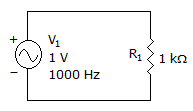# Electronics - Alternating Current vs Direct Current

### Exercise :: Alternating Current vs Direct Current - General Questions

11.

Which percentages of full-amplitude rise time are used for a pulse wave?

 A. 0 to 50 percent B. 0 to 100 percent C. 5 to 95 percent D. 10 to 90 percent

Answer: Option D

Explanation:

No answer description available for this question. Let us discuss.

12.

What is the average value of a 12 V peak wave?

 A. 3.82 V B. 4.24 V C. 7.64 V D. 9.42 V

Answer: Option C

Explanation:

No answer description available for this question. Let us discuss.

13.

A test equipment item that has the ability to produce either square, triangular, or sawtooth waveforms is called:

 A. a function generator B. a radio frequency generator C. an audio frequency generator D. a frequency meter or counter

Answer: Option A

Explanation:

No answer description available for this question. Let us discuss.

14.

The current is flowing in what direction?A. clockwise B. counterclockwise C. in both directions at the same time D. 50% of the time clockwise and 50% of the time counterclockwise

Answer: Option D

Explanation:

No answer description available for this question. Let us discuss.

15.

What does the CRT oscilloscope display?

 A. voltage and period B. current and frequency C. rms voltage and current D. frequency and voltage

Answer: Option A

Explanation:

No answer description available for this question. Let us discuss.

#### Current Affairs 2021

Interview Questions and Answers